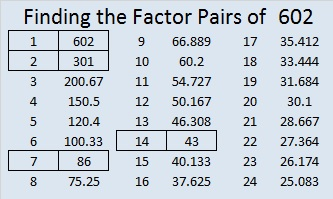# 602 and Level 1

(5^602) is a very large number with 421 digits. What are the first three of those 421 digits? 602. Thank you, OEIS.org for that interesting number fact.Print the puzzles or type the solution on this excel file: 10 Factors 2015-09-01

—————————————————————————————————

• 602 is a composite number.
• Prime factorization: 602 = 2 x 7 x 43
• The exponents in the prime factorization are 1, 1, and 1. Adding one to each and multiplying we get (1 + 1)(1 + 1)(1 + 1) = 2 x 2 x 2 = 8. Therefore 602 has exactly 8 factors.
• Factors of 602: 1, 2, 7, 14, 43, 86, 301, 602
• Factor pairs: 602 = 1 x 602, 2 x 301, 7 x 86, or 14 x 43
• 602 has no square factors that allow its square root to be simplified. √602 ≈ 24.535688.—————————————————————————————————This site uses Akismet to reduce spam. Learn how your comment data is processed.